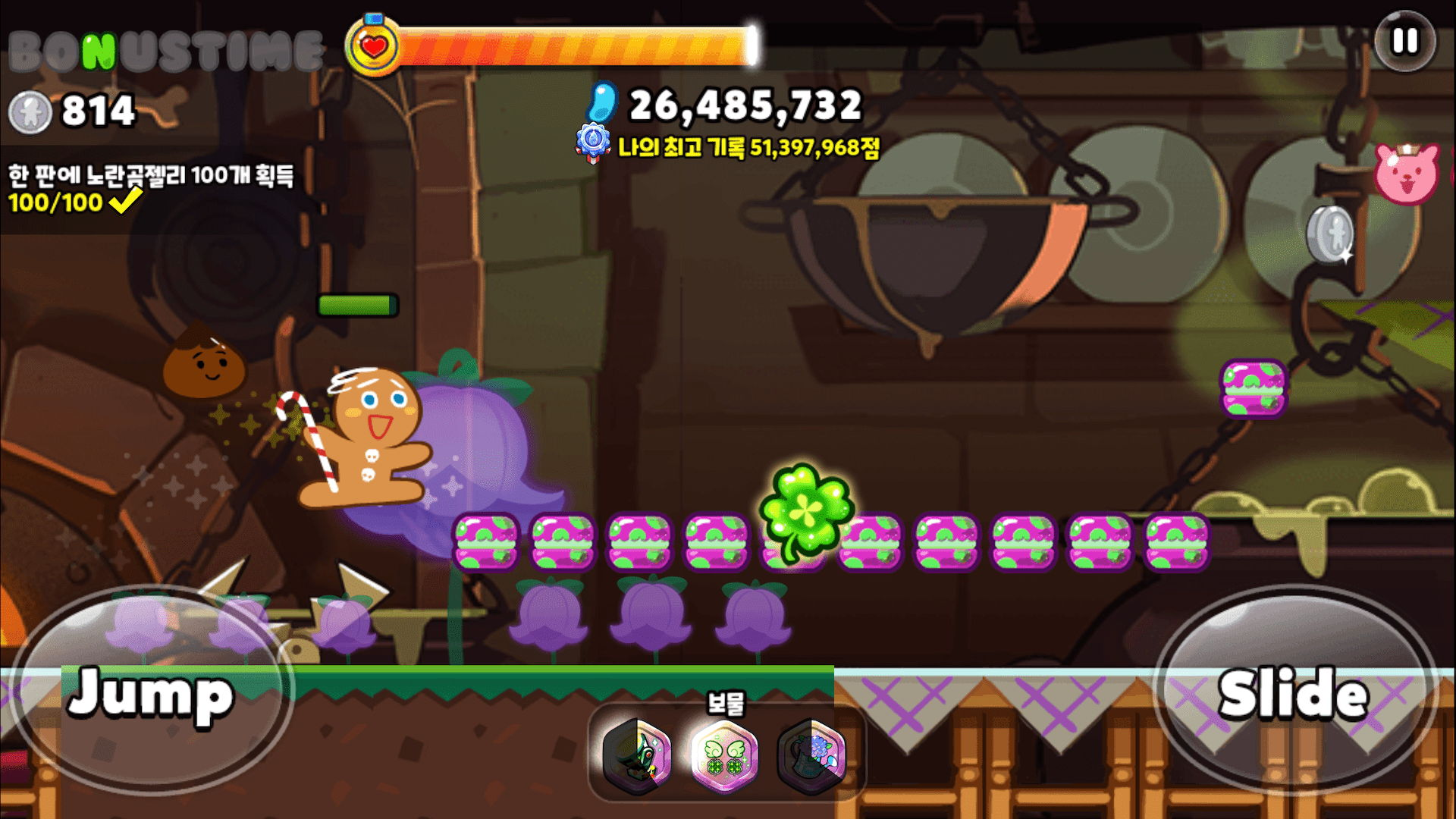시간 제한메모리 제한제출정답맞힌 사람정답 비율
2 초 1024 MB64353470.833%

## 문제

Cookie Run game is a popular game in which the cookie character runs through a map consisting of N stages to score points.Soo Young, the map designer for Cookie Run, was preparing a new map patch for Children's Day. After working hard to create an attractive map and testing it for the last time the day before the patch, she suddenly realized that the map was designed to be too easy!

The map design of the cookie run game consists of the following rules.

• Each stage is given a difficulty level of $A_0, A_1, A_2, ..., A_{N-1}$, and the higher the difficulty is, the more difficult it is to pass the corresponding stage.
• If the sum of the difficulty levels of consecutive stages is greater than or equal to $M$, we call this section as interesting section. That is, if $A_i + A_{i+1} + ... + A_j \geq M$, section $(i, j)$ is an interesting section.
• Maps should always have at least $K$ interesting section.

In other words, the following conditions should be satisfied:

• Let $T$ be the number of pairs $(i, j)$ satisfying the following conditions:
• $0 \le i \le j \le N-1$
• $A_{i} + A_{i+1} + ... + A_{j} \ge M$.
• Then, $T \ge K$ for the given integer $K$.

To solve this problem, Soo Young requested help from KAIST RUN Spring Contest participants.

Since there is not much time left until the patch release, all Soo Young can do is add difficulty $X$ to all stages at once to make it more difficult.

Your job is to find the smallest non-negative integer $X$ that satisfies these conditions.

## 입력

The first line contains three space-separated integers, $N$, $M$, and $K$.

The second line contains $N$ space-separated integers $A_{0}, A_{1}, \cdots, A_{N-1}$.

## 출력

Print a single non-negative integer denoting the smallest possible $X$.

You can assume that at least one non-negative integer $X$ exists, satisfying the given conditions.

## 제한

• $1 \le N \le 100\,000$
• $1 \le M\le 10^{18}$
• $1 \le K \le \frac{N (N+1)}{2}$
• $0 \le A_{i} \le 10^9$ $(0 \le i \le N-1)$

## 서브태스크 1 (10점)

• Smallest possible $X \le 100$
• $N \le 1\,000$

## 서브태스크 2 (20점)

• Smallest possible $X \le 100$
• $N \le 30\,000$

## 서브태스크 3 (30점)

• $N \le 5\,000$

## 예제 입력 1

3 20 5
4 0 4


## 예제 출력 1

16


## 예제 입력 2

5 30 4
1 2 3 4 5


## 예제 출력 2

6


## 예제 입력 3

4 32 4
8 7 5 9


## 예제 출력 3

9


## 채점 및 기타 정보

• 예제는 채점하지 않는다.